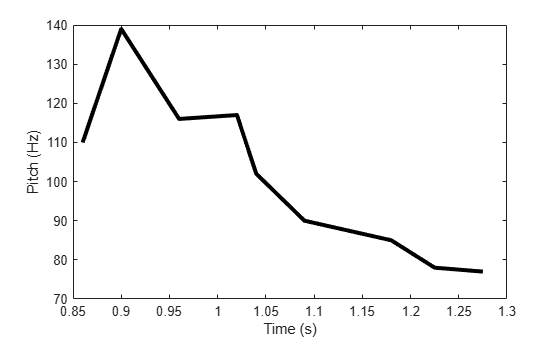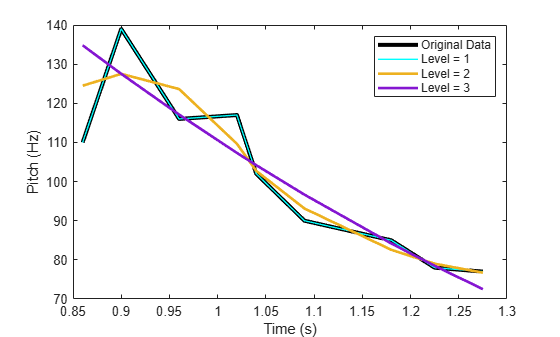# mlptrecon

Reconstruct signal using inverse multiscale local 1-D polynomial transform

## Syntax

``y = mlptrecon(type,coefs,T,coefsPerLevel,scalingMoments,reconstructionLevel)``
``y = mlptrecon(___,Name,Value)``

## Description

example

````y = mlptrecon(type,coefs,T,coefsPerLevel,scalingMoments,reconstructionLevel)` returns an approximation to the inverse multiscale 1-D polynomial transform (MLPT) of `coefs`.```

example

````y = mlptrecon(___,Name,Value)` specifies `mlptrecon` properties using one or more `Name,Value` pair arguments and the input arguments from the previous syntax.```

## Examples

collapse all

Create a low-frequency signal with high-frequency blips.

```t = (0:0.01:10)'; x = sin(2*pi.*t) + 0.5*sin(pi.*t+0.1); bliptime = (0:0.01:0.5)'; n = numel(bliptime); z0 = 2*(1:(n+1)/2)/(n+1); trng = [z0 z0((n-1)/2:-1:1)]'; blip = sin(50*pi.*bliptime).*trng; for i = [200,700,900] x(i:i+numel(bliptime)-1) = x(i:i+numel(bliptime)-1)+blip; end```

Perform a multilevel polynomial transform. Perform the inverse multilevel polynomial transform using the detail coefficients.

```[w,t,nj,scalingmoments] = mlpt(x,t); yDetails = mlptrecon('d',w,t,nj,scalingmoments,1);```

Plot the original signal and the processed signal.

```subplot(2,1,1) plot(t,x) title('Original Signal') subplot(2,1,2) plot(t,yDetails) title('Signal Details')```Approximate data using multiscale local polynomial transform (MLPT) reconstruction. Use `mlptrecon` to approximate a corrupted and sparsely sampled pitch contour.

Load input data and visualize it.

```load('CorruptedPitchData.mat'); plot(time,pitchContour,'k','linewidth',3) hold on xlabel('Time (s)') ylabel('Pitch (Hz)')```Compute the MLPT of the pitch contour.

```[w,t,nj,scalingMoments] = mlpt(pitchContour,time, ... 'DualMoments',3, ... 'PrimalMoments',4, ... 'PreFilter','none');```

Use `mlptrecon` to reconstruct the signal using the approximation coefficients at different levels.

```y = zeros(numel(t),3); for level = 1:3 y(:,level) = mlptrecon('a',w,t,nj,scalingMoments,level,'DualMoments',3); end```

Plot the reconstructed signals. Level two obtains the best smoothed estimate.

```plot(t,y(:,1),'c','linewidth',1) plot(t,y(:,2),'linewidth',2) plot(t,y(:,3),'linewidth',2) legend('Original Data','Level = 1','Level = 2','Level = 3') hold off```## Input Arguments

collapse all

Type of coefficients used to reconstruct the signal, specified as `'a'` or `'d'`.

• `'a'` — Approximation coefficients

• `'d'` — Detail coefficients

Approximation coefficients are a lowpass representation of the input. At each level, the approximation coefficients are divided into coarser approximation and detail coefficients.

Data Types: `char` | `string`

MLPT coefficients, specified as a vector or matrix of MLPT coefficients returned by the `mlpt` function.

Data Types: `double`

Sampling instants corresponding to `y`, specified as a vector or `duration` array of increasing values returned by the `mlpt` function.

Data Types: `double` | `duration`

Coefficients per resolution level, specified as a vector containing the number of coefficients at each resolution level in `coefs`. `coefsPerLevel` is an output argument of the `mlpt` function.

The elements of `coefsPerLevel` are organized as follows:

• `coefsPerLevel(1)` — Number of approximation coefficients at the coarsest resolution level.

• `coefsPerLevel(i)` — Number of detail coefficients at resolution level `i`, where ```i = numLevel – i + 2``` for ```i = 2,..., numLevel + 1```. `numLevel` is the number of resolution levels used to calculate the MLPT. `numLevel` is inferred from `coefsPerLevel`: ```numLevel = length(coefsPerLevel-1)```.

The smaller the index `i`, the lower the resolution. The MLPT is two times redundant in the number of detail coefficients, but not in t the number of approximation coefficients.

Data Types: `double`

Scaling function moments, specified as a `length(coefs)`-by-`P` matrix, where `P` is the number of primal moments specified by the MLPT.

Data Types: `double`

Resolution level used for reconstruction, specified as a positive integer less than or equal to `length(coefsPerLevel-1)`. `length(coefsPerLevel-1)` is the number of resolution levels used to calculate the MLPT. Increasing the value of `reconstructionLevel` corresponds to reconstructing your signal with coarser resolution approximations.

Data Types: `double`

### Name-Value Arguments

Specify optional pairs of arguments as `Name1=Value1,...,NameN=ValueN`, where `Name` is the argument name and `Value` is the corresponding value. Name-value arguments must appear after other arguments, but the order of the pairs does not matter.

Before R2021a, use commas to separate each name and value, and enclose `Name` in quotes.

Example: `'DualMoments',3` computes a transform using three dual vanishing moments.

Number of dual vanishing moments in the lifting scheme, specified as the comma-separated pair consisting of `'DualMoments'` and `2`, `3` or `4`. The number of dual moments must match the number used by `mlpt`.

Data Types: `double`

## Output Arguments

collapse all

Reconstructed approximation or details of signal, returned as a vector or matrix, depending on the inputs to the `mlpt` function.

Data Types: `double`

## Algorithms

Maarten Jansen developed the theoretical foundation of the multiscale local polynomial transform (MLPT) and algorithms for its efficient computation . The MLPT uses a lifting scheme, wherein a kernel function smooths fine-scale coefficients with a given bandwidth to obtain the coarser resolution coefficients. The `mlpt` function uses only local polynomial interpolation, but the technique developed by Jansen is more general and admits many other kernel types with adjustable bandwidths .

 Jansen, Maarten. “Multiscale Local Polynomial Smoothing in a Lifted Pyramid for Non-Equispaced Data.” IEEE Transactions on Signal Processing 61, no. 3 (February 2013): 545–55. https://doi.org/10.1109/TSP.2012.2225059.

 Jansen, Maarten, and Mohamed Amghar. “Multiscale Local Polynomial Decompositions Using Bandwidths as Scales.” Statistics and Computing 27, no. 5 (September 2017): 1383–99. https://doi.org/10.1007/s11222-016-9692-8.

 Jansen, Maarten, and Patrick Oonincx. Second Generation Wavelets and Applications. London ; New York: Springer, 2005.